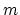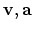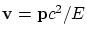Next: The notion of the center of masses Previous: Notions of relativistic dynamics   Contents

## The concept of mass

Now we proceed to more specific dynamical concepts. We begin with the concept of "mass". In order to introduce correctly the new physical concept of the "mass of a moving body" into SRT, it is necessary, first, to determine the procedure of measuring similar moving masses independently of any theory. (A similar procedure in GRT relates to the "mass of a body in the gravitational field": the distinction of the gravitation mass from inert one, as contrary to its own postulate). And this procedure should be namely the measurement, rather than re-calculation, for example, via the postulated formula for energy or momentum again. Otherwise the theory will try to "retain itself by the hair". A similar measurement procedure does not exist for SRT.

The physical concept of "mass" has no direct relation to all those formulas (it is mathematics), which can include letter "". For the basis concept of mass there exists the only clear - standard definition. It determines the mass just at the state of rest (for example, the conditions also exist for the standard of length - the temperature ones). And there is no need to "invent a bicycle". In the motion the mass is simply not defined, though lettercan enter quite diverse formulas containing, etc. These are different things! Therefore, the definition of an elementary concept of mass in terms of more complicatedly defined concepts of energy and momentum (depending on the theory, interpretation, state of a system, etc.) is a physical nonsense (though, possibly, it is correct mathematically). In such a manner one can "reach" an absurd and define a simple notion of velocity as. Note that any experiment, including measurement one, should be extremely clear defined with respect to all conditions of its performing. And, generally speaking, the "explanations" and "definitions" of theoretical physics (for example, in SRT) often represent by themselves a drop-out from physical understanding and a science-like masking of the essence of quantities behind (often correct) mathematical transformations.Next: The notion of the center of masses Previous: Notions of relativistic dynamics   Contents
Sergey N. Arteha